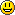# 5. Expected value

Let the probability distribution of a nonstandard two-sided coin toss be as follows

 $A$ $\text{Pr}[A]$ $\text{"heads on top"}$ $\text{"tails on top"}$ $\frac{2}{3}$ $\frac{1}{3}$

Let us have a game. If the coin shows heads, the bank will give you $2$ € and if the coin shows tails, you have to give to the bank $3$ €. Thus, we map all the results of the coin toss to real numbers:
$\text{"heads on top"}\longmapsto 2$,
$\text{"tails on top"}\longmapsto -3$,
and hence, create a random variable.

A random variable is a function $X:\Omega\longrightarrow\mathbb{R}$ that maps each elementary event $\omega\in\Omega$ to a real value $X(\omega)$.

In our examples $\Omega=\{\text{"heads on top"},\text{"tails on top"}\}$, and $X(\text{"heads on top"})=2$ and $X(\text{"tails on top"})=-3$.

Note, that we do not have to map each elementary event to a different number, i.e. we could map both heads and tails equal to $2$, but that would be a very boring game.

The probability distribution of the coin toss provides probability distribution also to the random variable:

 $x$ $\text{Pr}[X(\omega)=x]$ $2$ € $-3$ € $\frac{2}{3}$ $\frac{1}{3}$

Find the probability distribution of the random variable $Y$ defined by the following algorithm

y = 0
for i in range(1, 4)
x <- {0, 1}
y = y + x
return y

where $\text{x}$ is evaluated randomly by either $1$ or $0$.

Now, what amount of money you may expect to win with each game, if the coin is tossed several times? Indeed, this would be good information to know before entering the game, since you do want to know your risk.

In order to answer the question, you need the definition of expected value.

Let us have a random variable $X$:

 $\text{Pr}[X=x_i]$ $x_1$ $x_2$ $\quad\dots\quad$ $x_n$ $p_1$ $p_2$ $\quad\dots\quad$ $p_n$

Then the expected value $E(X)$ of $X$ is defines as

$$E(X)=x_1p_1+x_2p_2+\dots +x_np_n.$$

The expected value of a random variable describes the average value of the random variable. The probabilities $p_1,\dots,p_n$ can be viewed as weights, and since $p_1+\dots+p_n=1$, the formula of expected value is that of the weighted average: $$E(X)=\frac{x_1p_1+\dots+x_2p_n}{p_1+\dots+p_n}.$$

Now, in the case of the game, $$E(X)=2\cdot\frac{2}{3}+(-3)\cdot\frac{1}{3}=\frac{1}{3},$$ i.e., you average win is $33$ cents.

Can it be?! Just in case, check the result also in realityWrite a program in I Python which models the game. E.g. generate a random number $x$ between $0$ and $1$ (this is the coin flip). If $x<\frac{2}{3}$ then you win $2$ €, else you loose $3$ €. Flip the coin for example 1000 times and calculate the average of the sums.

Intuitively, expected value is the value you would obtain if you repeat the experiment/process with random results infinitely many times and find the average of the obtained results.

The roulette game consists of a small ball and a wheel with 38 numbered pockets around the edge. As the wheel is spun, the ball bounces around randomly until it settles down in one of the pockets.

One number is chosen by the player and a $2$ € bet is set on the number. Now, if the ball in the wheel settles on the same number, the player wins $30$ €, otherwise the player looses the bet. What is the expected profit from such a bet?

For example, if we wish to know the average value of a dice roll, we compute $$E(X)=1\cdot\frac{1}{6}+2\cdot\frac{1}{6}+3\cdot\frac{1}{6}+4\cdot\frac{1}{6}+5\cdot\frac{1}{6}+1\cdot\frac{1}{6}=\frac{7}{2}.$$ Let us have two dices and their corresponding random variables $X$ and $Y$. We roll the dices together. What is now the average value? Of course, we can always use the definition $E(X+Y)=(1+1)\frac{1}{36}+(1+2)\frac{1}{36}+\dots+(2+1)\frac{1}{36}+(2+2)\frac{1}{36}+\dots+(6+1)\frac{1}{36}+(6+2)\frac{1}{36}+\dots$ but more reasonable would be to use one of the basic properties of expected value.

For any random variable $X$ and $Y$, and any real-number $c$, we have:

• $E(c\cdot X)=c\cdot E(X)$.
• $E(X+Y)=E(X)+E(Y)$.
• If $\text{Pr}[X\leq Y]=1,$ then $E(X)\leq E(Y)$.

Thus, for two dices $E(X+Y)=E(X)+E(Y)=\frac{7}{2}+\frac{7}{2}=7$.

We roll a fair die $X$. What is $E[X^2]$?

You roll two fair die $X, Y$. You get $P:=X^2+Y$ points. What is $E[P]$?

Hint: use that you already know $E[X^2]$ and $E[Y]$.

You roll one fair die $X$. You get $P:=X^2+X$ points. What is $E[P]$?

Let $k\in\{1,\dots,2^n\}$ be a particular secret key. An adversary wishes to find out the secret key. All he can do is to try out keys and see whether he got the right one or not. The adversary tries all keys systematically (i.e., first he tries $k=1$, then $k=2$, $\dots$). Let $t$ denote the number of tries he makes. What is the average number of tries he needs in order to get the correct key?

An alternative way to compute an expected values (note the restrictions!) is by using the following formula.

Let $X$ be a random variable with values from $\mathbb{N}$, then $$E(X)=\sum_{i=1}^{\infty}\text{Pr}[X(\omega)\geq i].$$

In order to understand this formula, let us view a random variable $X$ given by the following probability distribution

 $x$ $\text{Pr}[X(\omega)=x]$ $1$ $2$ $3$ $4$ $5$ $p_1$ $p_2$ $p_3$ $p_4$ $p_5$

Now, let us draw lines with the length $p_1,\dots,p_5$ in one row

and put on each line segment a box with height of the corresponding $x$

We can calculated the area of all the rectangles

and find the whole area of this shape $1\cdot p_1+2\cdot p_2+3\cdot p_3+4\cdot p_4+5\cdot p_5$, which is exactly the expected value according to definition.

Actually, we can find the area of this shape also in another way:

i.e. $E(X)=1\cdot p_5+1\cdot(p_4+p_5)+1\cdot(p_3+p_4+p_5)+1\cdot(p_2+p_3+p_4+p_5)+1\cdot(p_1+p_2+p_3+p_4+p_5)$.

Note, that
$1\cdot p_5=\text{Pr}[X=5]=\text{Pr}[X\geq 5]$
$1\cdot (p_4+p_5)=\text{Pr}[X=4]+\text{Pr}[X=5]=\text{Pr}[X\geq 4]$
$1\cdot (p_3+p_4+p_5)=\text{Pr}[X=3]+\text{Pr}[X=4]+\text{Pr}[X=5]=\text{Pr}[X\geq 3]$
and so on. Thus,
$E(X)=\text{Pr}[X\geq 5]+\text{Pr}[X\geq 4]+\text{Pr}[X\geq 3]+\text{Pr}[X\geq 2]+\text{Pr}[X\geq 1]= \sum_{i=1}^{5}\text{Pr}[X\geq i]$.

You throw a die until you roll one. What is the expected number of tries?

Hint: first compute $\text{Pr}[\text{"the number of tries is$i$"}]$ for all $i$.